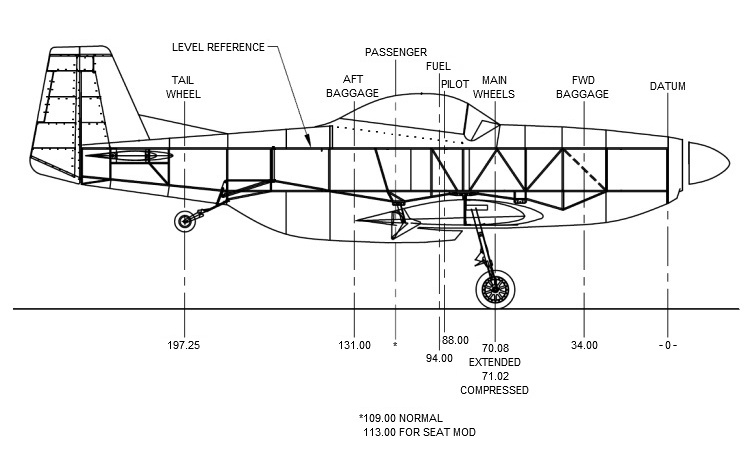spider

## Center of Gravity Calculator

 * Very Important - Figure Weight and Balance Before Flight * The moment is measured in inch pounds, where the inch dimension is the ARM length and pounds are the force (weight) applied at the ARM location. The datum -0- is the point from which all ARM lengths are measured. For the airplane, the individual moments for each item are added to give the airplanes total moment (about datum -0-). Next the total moment is divided by the aircrafts flying weight, giving you the C.G. location. The flying weight will change for various pilot weights, passenger weights, and/or fuel amount. Sample Calculation: Perform weight and balance in no wind conditions with airplane leveled using fuselage tube shown as a level reference. Measure and record the weights of all three wheels. (Note: Aircraft should be in empty configuration and should be resting on the two main wheels and tail wheel in the level position at all times during the measuring process.) Now experiment with different loading conditions, for example: Aft C.G. - 200 lbs pilot, full fuel, no baggage or passenger. Forward C.G. - heavy pilot, no fuel, heavy passenger or baggage. Fill in weight of aircraft, pilot, passenger, baggage and fuel Multiply weight times ARM to get the individual moment Add up total flying weight Add up total moment Divide moment by weight to get C.G. Recalculate taking into account fuel comsumptionWeight X ARM = Moment
Tail Wheel X 197.25 =
Right Main X 70.08 =
Left Main X 70.08 =
Pilot X 88.00 =
Passenger X * * =
Fuel X 94.00 =
FWD Baggage X 34.00 =
AFT Baggage X 131.00 =
Total = Total =
C.G. Location = Moment = = " C.G.
Weight
Acceptable C.G. Range: 72.00" (Forward) to 81.00" (Aft)
• Do not leave any Weight or ARM numbers blank or the calculator will error out.
I.E. If you are not carrying any baggage, put a zero in the weight spot.
• Note: All dimensions are as follows: Weight are pounds, ARMs are inches,
Moments are inch pounds.